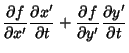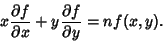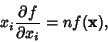## Euler's Homogeneous Function Theorem

Letbe a Homogeneous Function of orderso that(1)

Then defineand. Then(2)

Let, then(3)

This can be generalized to an arbitrary number of variables(4)

where Einstein Summation has been used.